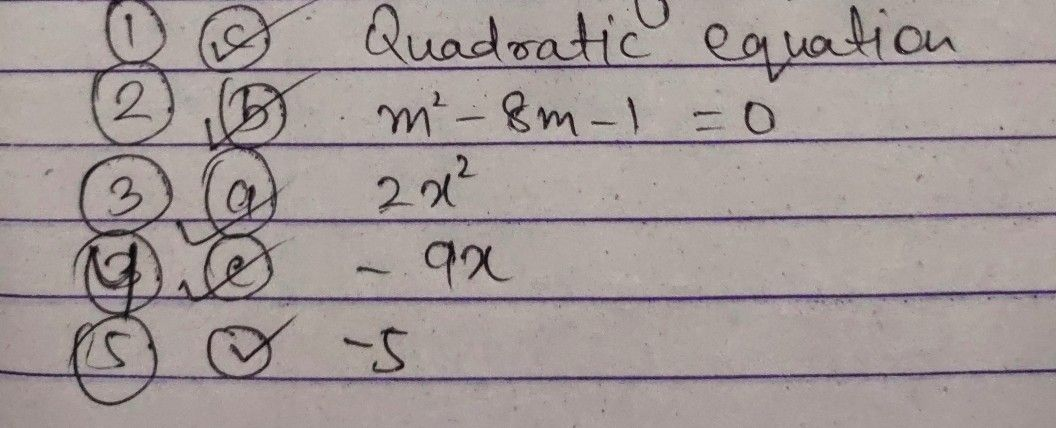Symbol
Problemletter that you think best answers the question. Piease answer all items Take hote of the items that you were not able to answer correctly and find the right answer as you go through this module. polynomial equation of degree two that can be written in the form 1. $11lsap0yn0mia|$ $ax^{2}+bx+c=0$ where a, b, and c are real numbers and $a+0$ $a$ Linear Equation c. Quadratic Equation b. Linear Inequality d. Quadratic Inequality 2. Which of the following is a quadratic equation? $a$ $3s^{2}+s-4$ c. $2x-1=5$ b. $m^{2}-8m-1=0$ d. $5y^{2}+4y\geq 7$ 3. In the quadratic equation $2x^{2}-9x-5=0$ which is the quadratic term? a. 2x2 $bx^{2}$ C.- 9x $4-5$ $on2x^{2}-9x-5=0$ $x^{2}$ which is the linear term? $4$ In the quadratic equation $a$ 2x b. $c-9x$ $d-5$ $5$ In the quadratic equation $2x^{2}-9x-5=0$ which is the constant term? 1.
1st-6th grade
Probability and Statistics
Search count: 135
SolutionQanda teacher - amir